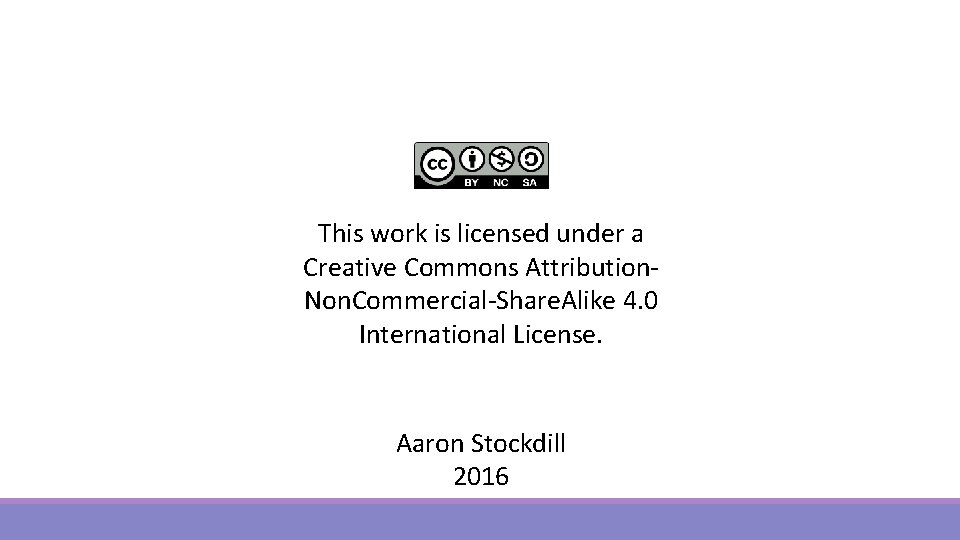# Trigonometry MAGIC TRIANGLES IDENTITIES Magic Triangles There are

• Slides: 26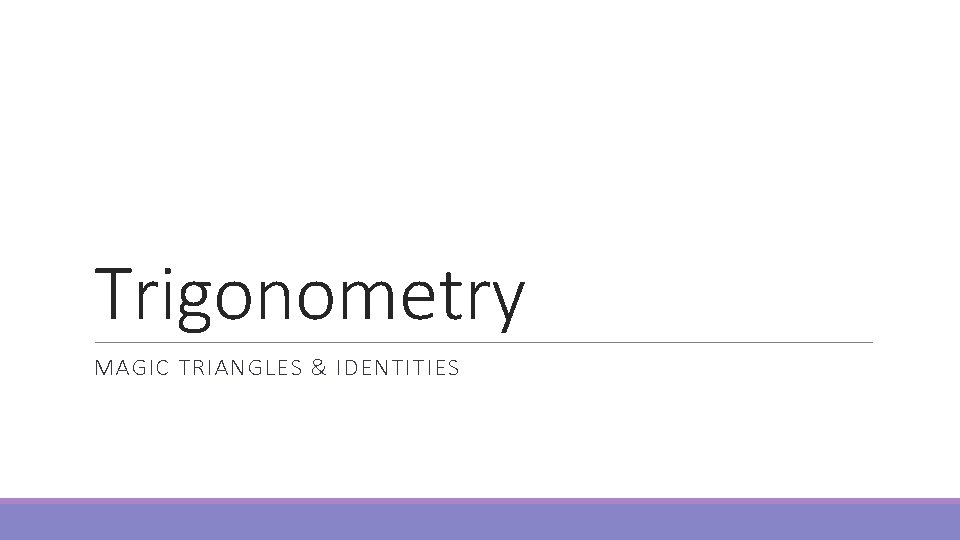Trigonometry MAGIC TRIANGLES & IDENTITIESMagic Triangles There are 3 magic triangles, two of which are on your formula sheet They let you do some trigonometric equations without calculators These are a fundamental part of ”exact solutions”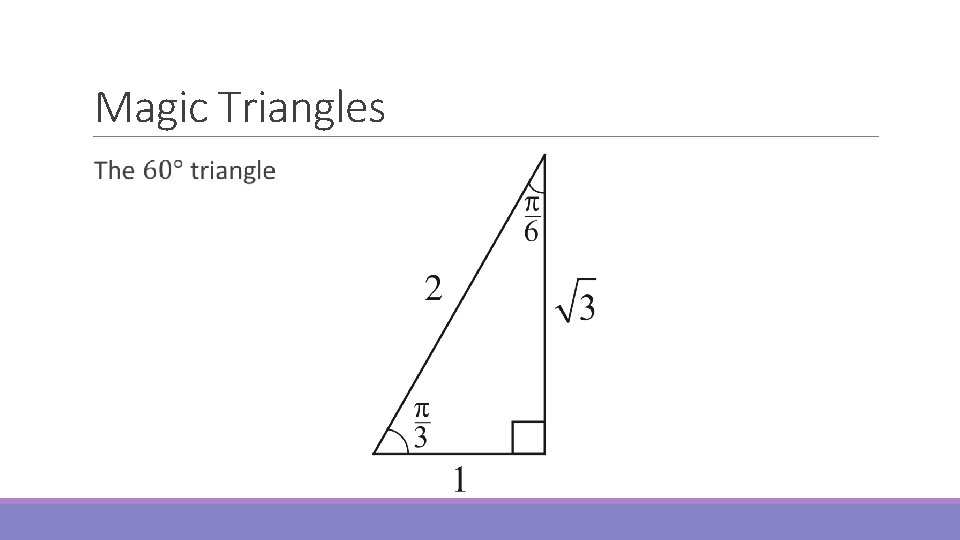Magic Triangles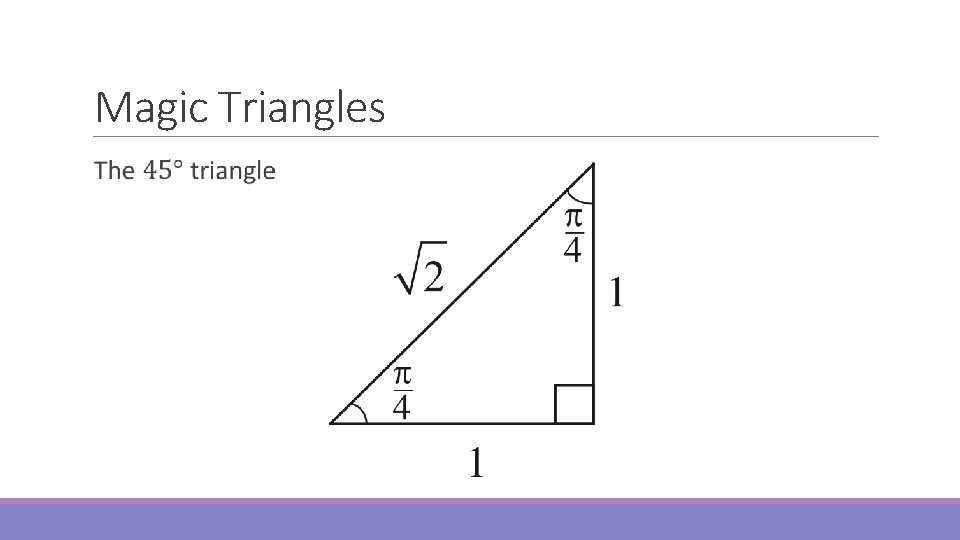Magic Triangles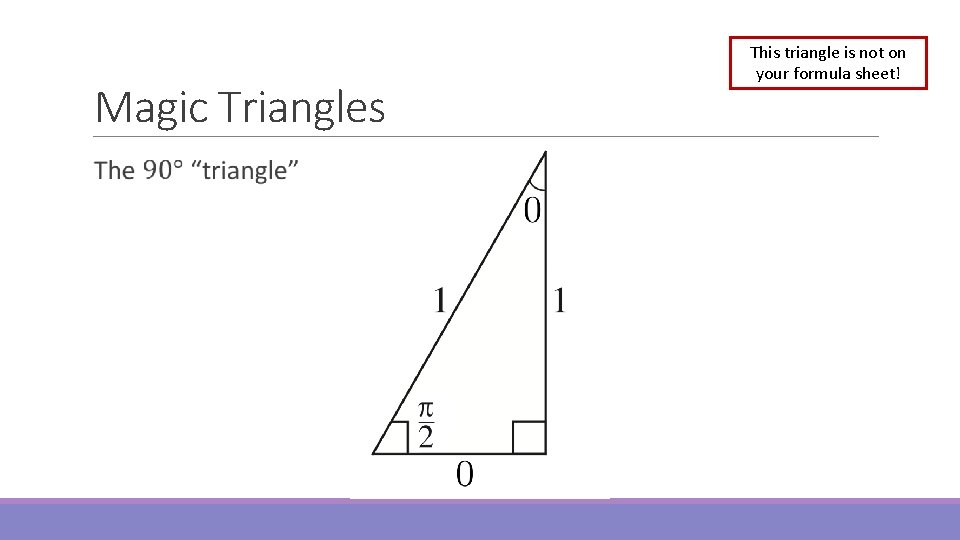Magic Triangles This triangle is not on your formula sheet!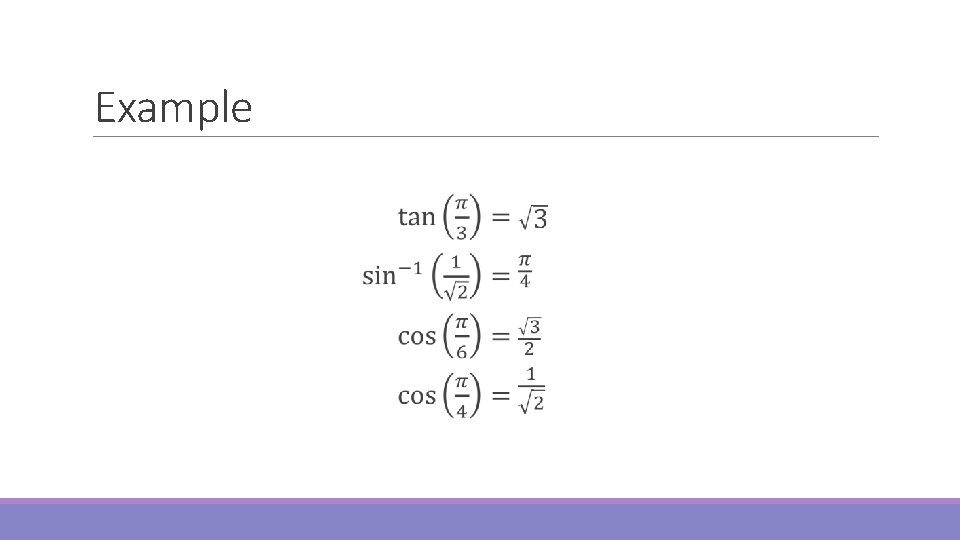ExampleExact SolutionsExact SolutionsExample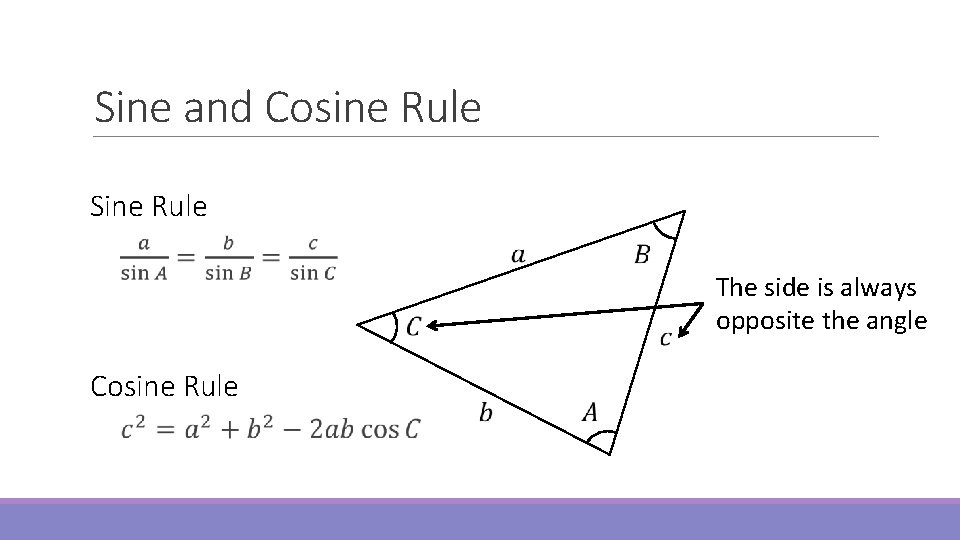Sine and Cosine Rule Sine Rule Cosine Rule The side is always opposite the angleSine and Cosine Rule When to use the Sine Rule: If you have at least one side-angle pair When to use the Cosine Rule: If you have 2 sides and the angle between them If you have all three sides and no angles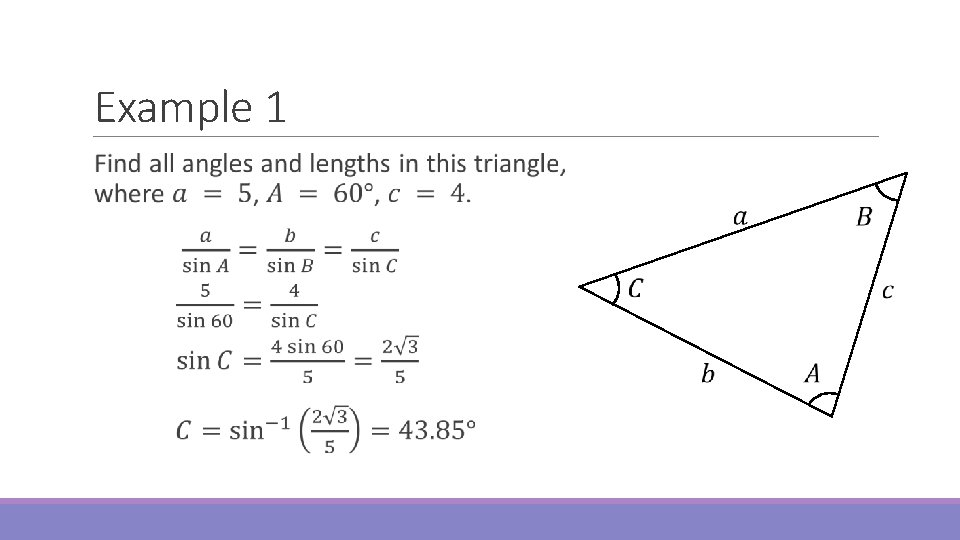Example 1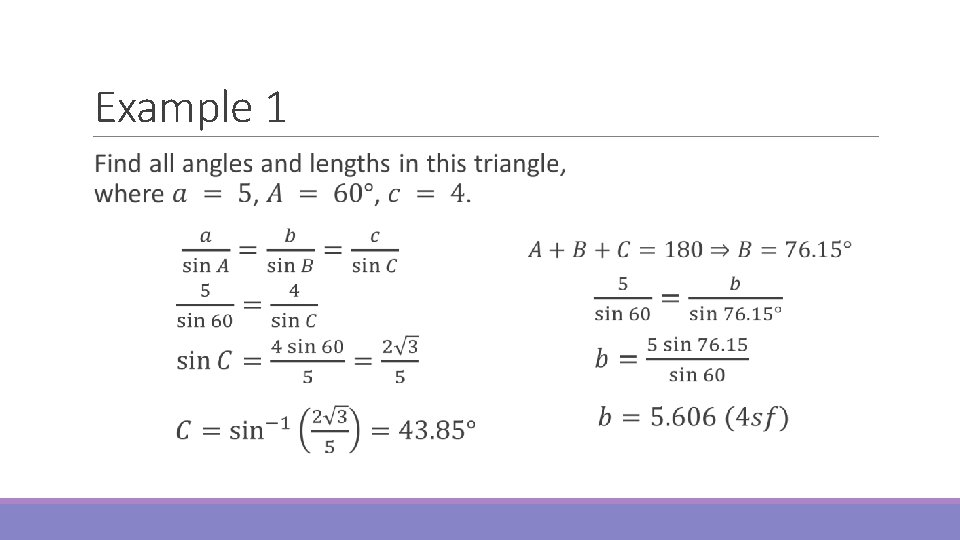Example 1Example 2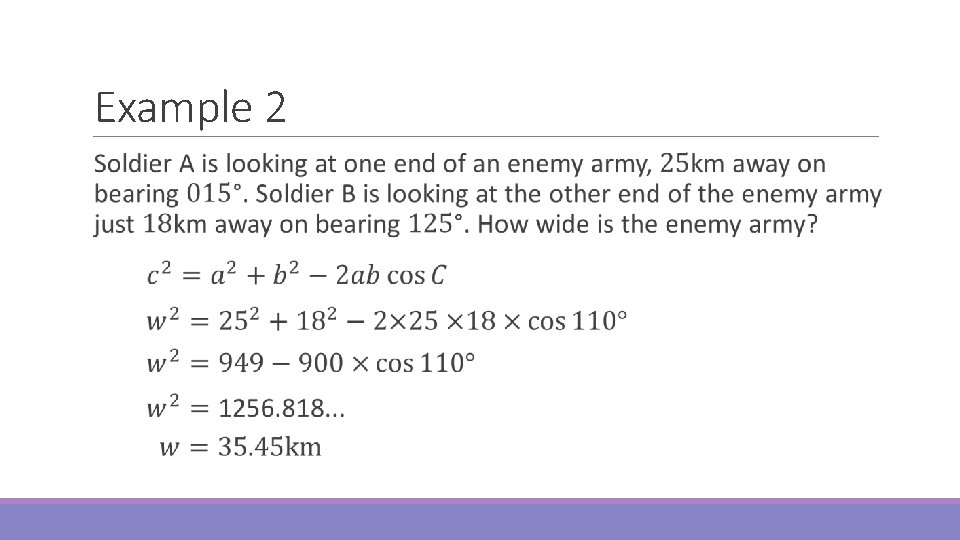Example 2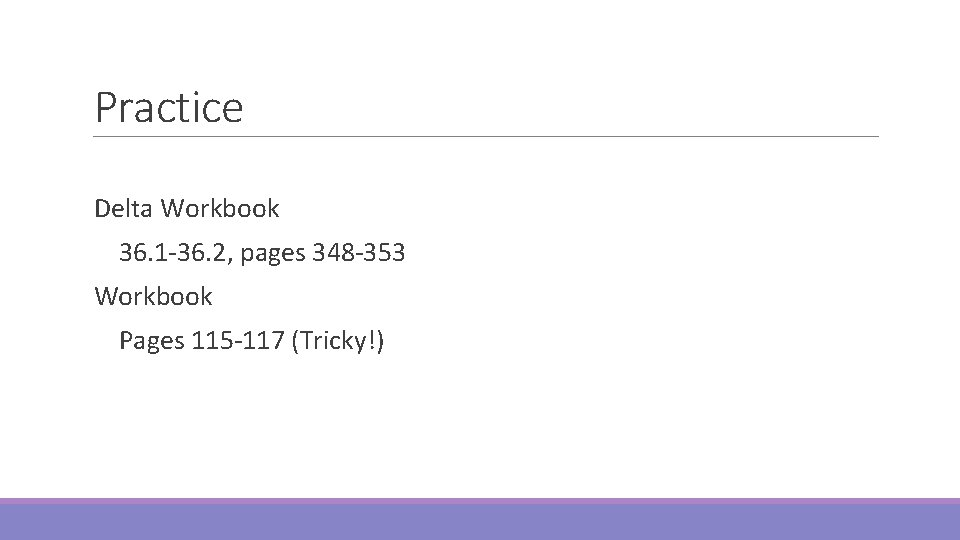Practice Delta Workbook 36. 1 -36. 2, pages 348 -353 Workbook Pages 115 -117 (Tricky!)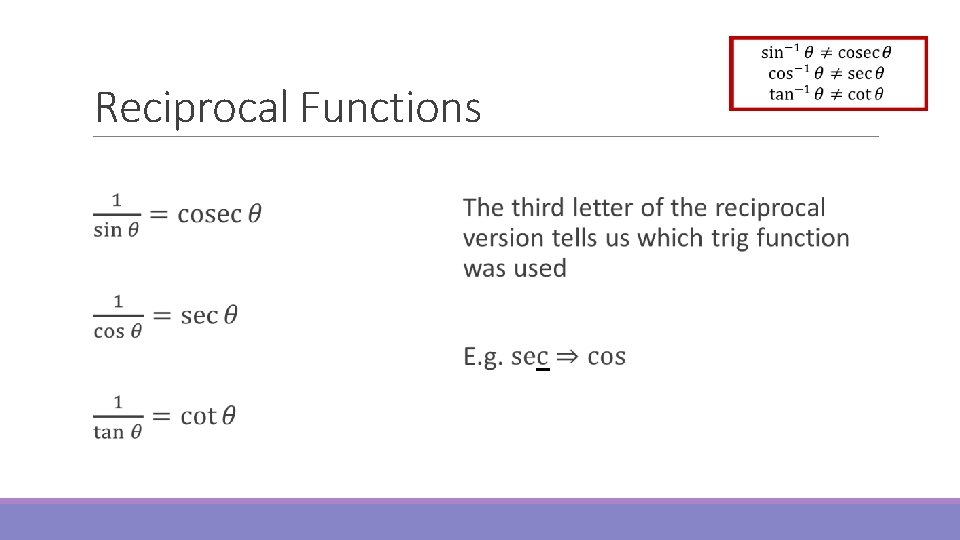Reciprocal FunctionsReciprocal Functions Not on the Formula Sheet!Trigonometric Identities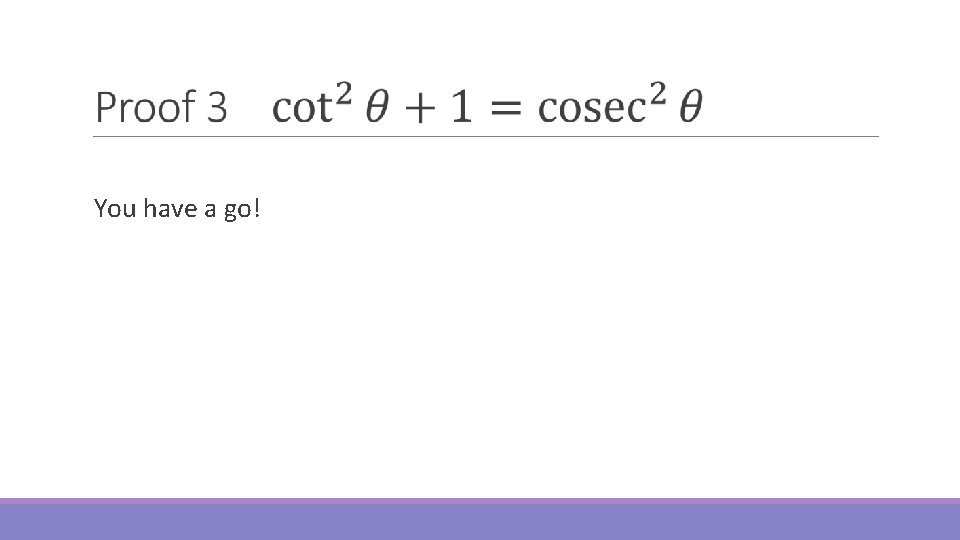You have a go!Practice Delta Workbook 33. 4, page 315 34. 1 -34. 2, pages 320 -321 Workbook Pages 92 -95Do Now Any Questions? Delta Workbook Exercises 33. 5, 34. 1 -34. 2, 36. 1 -36. 2 Workbook Pages 92 -95, 115 -117